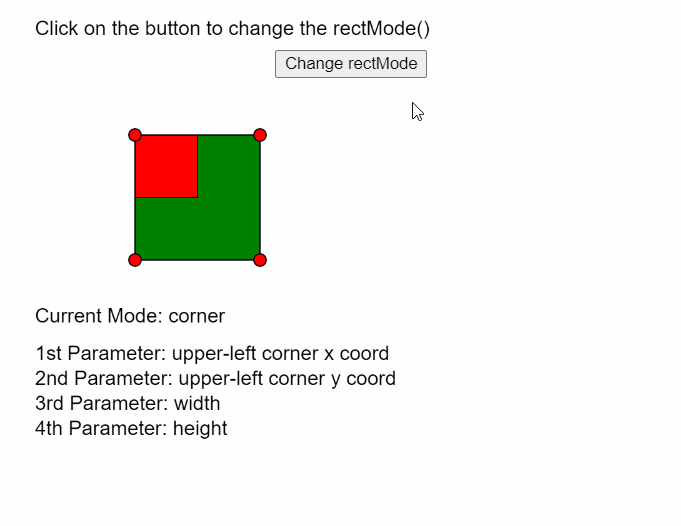GFG App
Open AppBrowser
Continue

# p5.js | rectMode() Function

The rectMode() function in p5.js is used to change the way in which the parameters given to the rect() function are interpreted. This modifies the location from where the rectangle is drawn.

This function can be given four modes:

• CORNER: This mode interprets the first two parameters as the upper-left corner of the shape. The third and fourth parameters are its width and height. It is the default mode.
• CORNERS:This mode interprets the first two parameters as the upper-left corner and the other two parameters as the location of the opposite corner.
• CENTER: This mode interprets the first two parameters as the shape’s center point. The third and fourth parameters specify the shape’s width and height.
• RADIUS:This mode interprets the first two parameters as the shape’s center point. The third and fourth parameters specify half of the shape’s width and height.

Syntax:

`rectMode( mode )`

Parameters: This function accepts a single parameter as mentioned above and described below.

• mode: It is a Constant which defines which mode to use. It can have the values of CORNER, CORNERS, CENTER, or RADIUS.

The examples below illustrate the rectMode() function in p5.js:

Example:

 `let currMode; ` ` `  `function` `setup() { ` `  ``createCanvas(500, 400); ` `  ``textSize(16); ` ` `  `  ``// Define all the rectModes() ` `  ``let rectModes = [CORNER, CORNERS, CENTER, RADIUS]; ` `  ``let index = 0; ` `  ``currMode = rectModes[index]; ` ` `  `  ``// Define a button to switch between the modes ` `  ``let closeBtn = createButton(``"Change rectMode"``); ` `  ``closeBtn.position(220, 40); ` `  ``closeBtn.mouseClicked(() => { ` `    ``if` `(index < rectModes.length - 1) index++; ` `    ``else` `index = 0; ` `    ``currMode = rectModes[index]; ` `  ``}); ` `} ` ` `  `function` `draw() { ` `  ``clear(); ` `  ``text(``"Click on the button to"``+ ` `       ``" change the rectMode()"``, 20, 20); ` `  ``fill(``"green"``); ` ` `  `  ``// Draw the first rectangle with default mode ` `  ``rectMode(CORNER); ` `  ``rect(100, 100, 100, 100); ` ` `  `  ``fill(``"red"``); ` ` `  `  ``// Set the rectMode according to the defined mode ` `  ``rectMode(currMode); ` ` `  `  ``// Draw the second rectangle according to the ` `  ``// selected rectMode() and different dimensions ` `  ``rect(100, 100, 50, 50); ` ` `  `  ``// Draw circles to demonstrate corners of ` `  ``// the first rectangle ` `  ``circle(100, 100, 10); ` `  ``circle(200, 100, 10); ` `  ``circle(100, 200, 10); ` `  ``circle(200, 200, 10); ` ` `  `  ``fill(``"black"``); ` `  ``text(``"Current Mode: "` `+ currMode, 20, 250); ` ` `  `  ``// Show details of parameter according to selected mode ` `  ``switch` `(currMode) { ` `    ``case` `CORNER: ` `      ``text(``"1st Parameter: upper-left"``+ ` `           ``" corner x coord"``, 20, 280); ` `      ``text(``"2nd Parameter: upper-left"``+ ` `           ``" corner y coord"``, 20, 300); ` `      ``text(``"3rd Parameter: width"``, 20, 320); ` `      ``text(``"4th Parameter: height"``, 20, 340); ` `      ``break``; ` `    ``case` `CORNERS: ` `      ``text(``"1st Parameter: upper-left corner"``+ ` `           ``" x coord"``, 20, 280); ` `      ``text(``"2nd Parameter: upper-left corner"``+ ` `           ``" y coord"``, 20, 300); ` `      ``text(``"3rd Parameter: opposite corner x"``, 20, 320); ` `      ``text(``"4th Parameter: opposite corner y"``, 20, 340); ` `      ``break``; ` `    ``case` `CENTER: ` `      ``text(``"1st Parameter: shape's center"``+ ` `           ``" point x coord"``, 20, 280); ` `      ``text(``"2nd Parameter: shape's center"``+ ` `           ``" point y coord"``, 20, 300); ` `      ``text(``"3rd Parameter: width"``, 20, 320); ` `      ``text(``"4th Parameter: height"``, 20, 340); ` `      ``break``; ` `    ``case` `RADIUS: ` `      ``text(``"1st Parameter: shape's center"``+ ` `           ``" point x coord"``, 20, 280); ` `      ``text(``"2nd Parameter: shape's center"``+ ` `           ``" point y coord"``, 20, 300); ` `      ``text(``"3rd Parameter: half of shape's"``+ ` `           ``" width"``, 20, 320); ` `      ``text(``"4th Parameter: half of shape's"``+ ` `           ``" height"``, 20, 340); ` `      ``break``; ` `    ``default``: ` `      ``break``; ` `  ``} ` `} `

Output:Online editor: https://editor.p5js.org/

Reference: https://p5js.org/reference/#/p5/rectMode

My Personal Notes arrow_drop_up